Courses

# Free & Forced Vibration Notes | EduRev

## Mechanical Engineering : Free & Forced Vibration Notes | EduRev

The document Free & Forced Vibration Notes | EduRev is a part of the Mechanical Engineering Course Theory of Machines (TOM).
All you need of Mechanical Engineering at this link: Mechanical Engineering

Mechanical Vibrations

The to and for motion of a body about its mean position is termed as mechanical vibrations or harmonic vibrations or oscillations.
[Mean Position = Equilibrium Position = Zero Position] All are same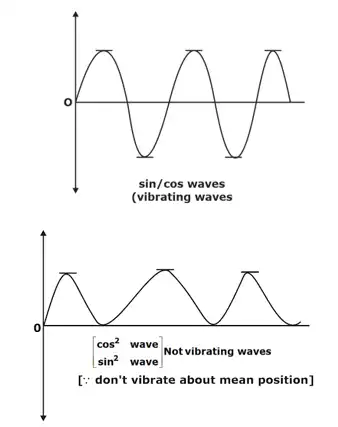Type of oscillating waves

Any vibrating system is a combination of:

• E storing device (i.e. mass = m)
• E storing device (having stiffness = s)
• Kinetic friction
• Unbalanced forces
1. Natural Vibrations
The vibrations in which there is no friction at all (static friction may have, but no kinetic friction) as well as there is no unbalanced force after the initial release of the system are called as “Natural Vibrations”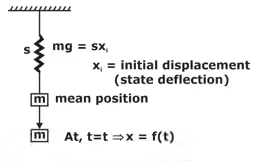Spring-mass systemUsing D-Alembert's Principle:
ma + sx = 0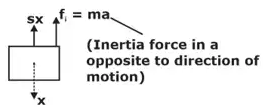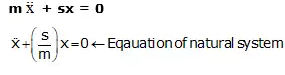2. Energy Method to Calculate Natural Frequency
This method is used only for natural vibrations and is used specially for Rolling problems.
In Natural Vibrations,
As, kinetic friction = 0
Total energy = constant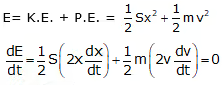3. Torsional Vibrations
The vibrations of a system about its own center of mass is termed as torsional vibrations.
In case of torsional vibrations, At fixed Print, as. Hence, Vibration’s amplitude will also zero. This point is called ‘Node’.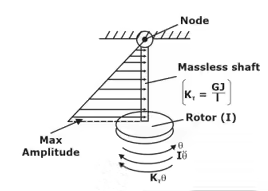shaft-rotor system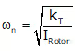(kT = Torsional stiffness of shaft)
Note: If shaft mass MOI is also considered (= Is)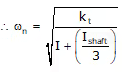(i) Two-Rotor System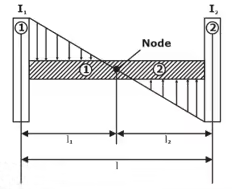2-rotor systemAt Node Point:
ωn1 = ωn2 (i.e. net vibrations of Node Point = 0)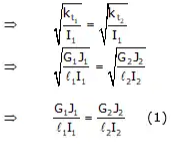for this same shaft, G1J1 = G2 J2      [∵ G1 = G2, J1 = J2]
⇒ I11 = I22    (2)
Also, ℓ1 + ℓ2 = ℓ   (3)
4. Rayleigh’s Method to Calculate Natural Frequency (Method of Static Deflection of Mass)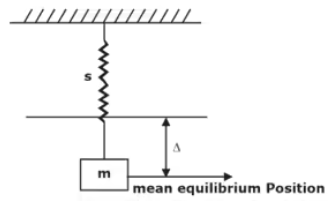Basic spring Mass System∇ = static deflection of mass ′m′
= (mg/s)  [∵ mg = s · ∇]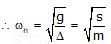5. Longitudinal-Vibrations of Beams
Vibrations along the length of Beam are termed as longitudinal vibrations.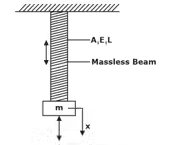longitudinal vibrations of a beamAxial or longitudinal stiffness of beam,
s = (AE/L)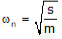6. Transverse –Vibrations of Beams
Vibrations in a direction perpendicular to axis of the Beam.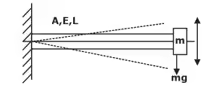Transverse vibrations of a beam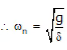where, δ = static defection of Beam Under transverses loads
Example:
For a cantilever Beam with Point load 'w' at free end δ = WL3/ 3EL
Offer running on EduRev: Apply code STAYHOME200 to get INR 200 off on our premium plan EduRev Infinity!

## Theory of Machines (TOM)

94 videos|41 docs|28 tests

,

,

,

,

,

,

,

,

,

,

,

,

,

,

,

,

,

,

,

,

,

;